Related Articles

# Mathematical Operations in Solidity

• Last Updated : 18 Sep, 2020

Solidity is a brand-new programming language created by the Ethereum which is the second-largest market of cryptocurrency by capitalization, released in the year 2015 led by Christian Reitwiessner. Ethereum is a decentralized open-source platform based on blockchain domain, used to run smart contracts i.e. applications that execute the program exactly as it was programmed without the possibility of any fraud, interference from a third party, censorship, or downtime

Smart contracts are high-level program codes that are compiled to EVM byte code and deployed to the Ethereum blockchain for further execution. It allows us to perform credible transactions without any interference of the third party, these transactions are trackable and irreversible. Mathematical operations in solidity are as simple as we use these operations in real life. There are following Mathematical Operations in Solidity:

2. Subtraction: (x – y)
3. Multiplication (x * y)
4. Division (x / y)
5. Modulus (x % y)
6. Exponential  (x**y)

In this article, we will be discussing these operations in detail with examples.

The addition operation involves adding the two numbers and generating a sum.

Example: In the below example, a contract is created with two functions to set the values of the two variables and a third function to add the two variables and return the sum.

## Solidity

 `// Solidity program to ``// demonstrate addition``pragma solidity 0.6.6;``contract` `gfgMathPlus ``{``    ``// Declaring the state``    ``// variables``    ``uint firstNo ;``    ``uint secondNo ;`` ` `    ``// Defining the function ``    ``// to set the value of the ``    ``// first variable``    ``function firstNoSet(uint x) ``public` `    ``{``        ``firstNo = x;``    ``}`` ` `    ``// Defining the function``    ``// to set the value of the ``    ``// second variable``    ``function secondNoSet(uint y) ``public` `    ``{``        ``secondNo = y;``    ``}`` ` `    ``// Defining the function``    ``// to add the two variables``    ``function add() view ``public` `returns (uint) ``    ``{``        ``uint Sum = firstNo + secondNo ;``         ` `        ``// Sum of two variables``        ``return` `Sum;``    ``}``}`

#### Output: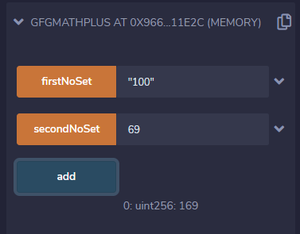### Subtraction

The subtraction operation involves subtracting the two numbers and generating a difference. In Subtraction, we could have used any value of Integer (int16 to int256) but all the operands must be of the same data types (same bits Integers).

Example: In the below example, a contract is created with a function to demonstrate the subtraction of two numbers.

## Solidity

 `// Solidity program to ``// demonstrate the subtraction``pragma solidity 0.6.6;``contract` `gfgSubract ``{``    ``// Intializing the``    ``// state variables``    ``int16 firstNo=2 ;``    ``int16 secondNo=10;``     ` `    ``// Defining a function``    ``// to subtract two numbers``    ``function Sub() view ``public` `returns (int16) ``    ``{``        ``int16 ans = firstNo - secondNo ;``         ` `        ``// Difference amount``        ``return` `ans;``    ``}`` ` `}`

#### Output: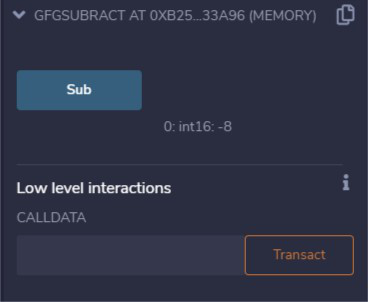### Multiplication

The multiplication operation deals with generating a product value by multiplying two or more numbers.

Example: In the below example, a contract is created with three functions, where the first two functions are responsible for setting the values of the variables, and the third function generates a product value by multiplying the first two variables.

## Solidity

 `pragma solidity 0.6.6;`` ` `contract` `gfgMultiply {`` ` `    ``int128 firstNo ;``    ``int128 secondNo ;``     ` `    ``function firstNoSet(int128 x) ``public` `{``        ``firstNo = x;``    ``}``     ` `    ``function secondNoSet(int128 y) ``public` `{``        ``secondNo = y;``    ``}``     ` `    ``function multiply() view ``public` `returns (int128) {``        ``int128 answer = firstNo * secondNo ;``        ``return` `answer;``    ``}`` ` `}`

#### Output: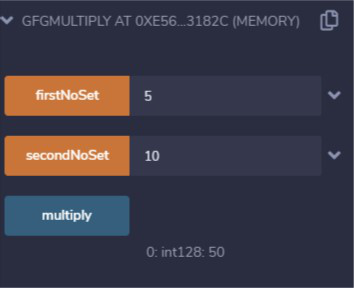### Division

The division operation returns the quotient of the division result.

Example: In the below example, a contract is created with three functions, where the first two functions are responsible for setting the values of the variables, and the third function generates a quotient value by dividing the second number by the first number.

## Solidity

 `// Solidity program to``// demontstrate the ``// division operation``pragma solidity 0.6.6;`` ` `// Creating a contract``contract` `gfgDivide ``{``    ``// Declaring the ``    ``// state variables``    ``int128 firstNo ;``    ``int128 secondNo ;``     ` `    ``// Defining a function``    ``// to set the value of ``    ``// the first variable``    ``function firstNoSet(int64 x) ``public` `    ``{``        ``firstNo = x;``    ``}``     ` `    ``// Defining function``    ``// to set the value of``    ``// the second variable``    ``function secondNoSet(int64 y) ``public` `    ``{``        ``secondNo = y;``    ``}``     ` `    ``// Defining function to``    ``// return the result``    ``function Divide() view ``public` `returns (int128) ``    ``{``        ``int128 answer = firstNo / secondNo ;``        ``return` `answer;``    ``}``}`

Note:

1. We can see that we divided two 64-bit Integers and successfully stored the result in a 128-bit Integer.
2. This is only possible in the case of Multiplication and Division Operation.

Output: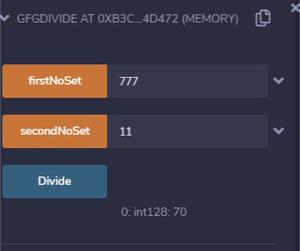### Modulus Or Remainder

This operation returns the remainder result of the process of division fo two numbers.

Example: In the below example, a contract is created with three functions, where the first two functions are responsible for setting the values of the variables, and the third function generates a remainder value by dividing the second number by the first number. In Modulo Operation all the variables must be Integers of the Same Bits Length.

## Solidity

 `// Solidity program to``// demonstrate the ``// Modulus operation``pragma solidity ^0.6.6;`` ` `// Creating a contract``contract` `gfgModulo ``{``    ``// Declaring state variables``    ``uint firstNo ;``    ``uint secondNo ;``     ` `    ``// Defining a function``    ``// to set the value of``    ``// the first variable``    ``function firstNoSet(uint x) ``public` `    ``{``        ``firstNo = x;``    ``}``     ` `    ``// Defining a function``    ``// to set the value of``    ``// the second variable``    ``function secondNoSet(uint y) ``public` `    ``{``        ``secondNo = y;``    ``}``     ` `    ``// Defining a function to return``    ``// the modulus value``    ``function Modulo() view ``public` `returns (uint) ``    ``{``        ``uint answer = firstNo % secondNo ;``        ``return` `answer;``    ``}``}`

#### Output: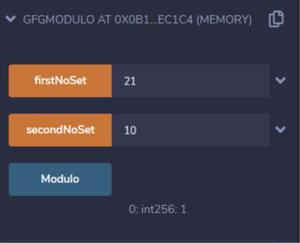### Exponentiation

The exponentiation operation only works on the Unsigned Integers where the lower bits unsigned Integers can be calculated and stored in a higher bit unsigned Integer.

## Solidity

 `// Solidity program to``// demonstrate the ``// exponentiation operation``pragma solidity ^0.6.6;`` ` `// Creating a contract``contract` `gfgExpo ``{``    ``// Declaring the state ``    ``// variables``    ``uint16 firstNo ;``    ``uint16 secondNo ;`` ` `    ``// Defining the first function``    ``// to set the value of ``    ``// first variable``    ``function firstNoSet(uint16 x) ``public` `    ``{``        ``firstNo = x;``    ``}``     ` `    ``// Defining the function``    ``// to set the value of ``    ``// the second variable``    ``function secondNoSet(uint16 y) ``public` `    ``{``        ``secondNo = y;``    ``}``     ` `    ``// Defining the function to``    ``// calculate the exponent``    ``function Expo() view ``public` `returns (uint256) {``        ``uint256 answer = firstNo ** secondNo ;``        ``return` `answer;``    ``}``}`

#### Output: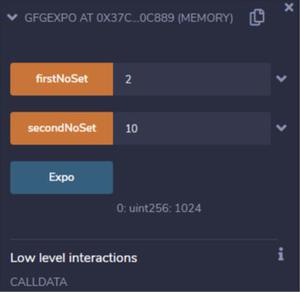#### Note:

1. In Addition, Subtraction, and Modulo all the operands must be the same size Integer.
2. In Division and Multiplication, the calculated answer can be stored in the greater bits Integer but the operands must be the same size Integer.
3. In the Exponentiation function, the operands must be unsigned Integer. Lower bits Unsigned Integers can be calculated and stored in a higher but Unsigned Integer.
4. You need to set the same data type of all the operands in order to apply a mathematical operation on them else they will not execute.

My Personal Notes arrow_drop_up